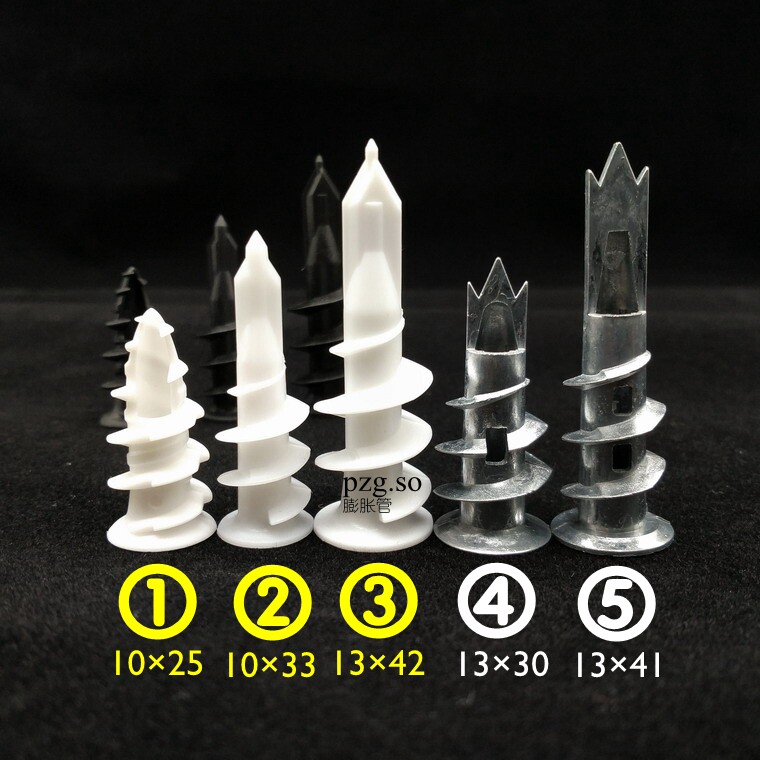# 尼小龙培训资料第5课：塑料螺丝的耐蚀性## 订购链接：Nxlon.com/1006

PA66相对耐热性=6，相对耐蚀性：有机溶剂=7，碱类=7，酸类=3，氧化=2

POM相对耐热性=7，相对耐蚀性：有机溶剂=9，碱类=3，酸类=3，氧化=3

PC 相对耐热性=8，相对耐蚀性：有机溶剂=6，碱类=1，酸类=7，氧化=6

ABS相对耐热性=3，相对耐蚀性：有机溶剂=4，碱类=8，酸类=9，氧化=4

PP 相对耐热性=3，相对耐蚀性：有机溶剂=5，酸类=10，氧化=8

PE 相对耐热性=1，相对耐蚀性：有机溶剂=5，碱类=10，酸类=10，氧化=8

PVC相对耐热性=4，相对耐蚀性：有机溶剂=6，碱类=10，酸类=10，氧化=6

PTFE相对耐热性=9，相对耐蚀性：有机溶剂=10，碱类=10，酸类=10，氧化=10

## 中山市尼小龙塑料制品有限公司

Zhongshan Nxlon Plastic Products Co.,Ltd.